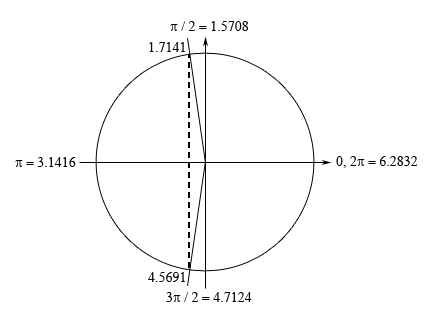Paul's Online Notes
Home / Calculus I / Review / Trig Equations with Calculators, Part I
Show Mobile Notice Show All Notes Hide All Notes
Mobile Notice
You appear to be on a device with a "narrow" screen width (i.e. you are probably on a mobile phone). Due to the nature of the mathematics on this site it is best views in landscape mode. If your device is not in landscape mode many of the equations will run off the side of your device (should be able to scroll to see them) and some of the menu items will be cut off due to the narrow screen width.

### Section 1.5 : Solving Trig Equations with Calculators, Part I

1. Find all the solutions to $$7\cos \left( {4x} \right) + 11 = 10$$. Use at least 4 decimal places in your work.

Show All Steps Hide All Steps

Hint : Isolate the cosine (with a coefficient of one) on one side of the equation.
Start Solution

Isolating the cosine (with a coefficient of one) on one side of the equation gives,

$\cos \left( {4x} \right) = - \frac{1}{7}$
Hint : Using a calculator and your knowledge of the unit circle to determine all the angles in the range $$\left[ {0,2\pi } \right]$$ for which cosine will have this value.
Show Step 2

First, using our calculator we can see that,

$4x = {\cos ^{ - 1}}\left( { - \frac{1}{7}} \right) = 1.7141$

Now we’re dealing with cosine in this problem and we know that the $$x$$-axis represents cosine on a unit circle and so we’re looking for angles that will have a $$x$$ coordinate of $$- \frac{1}{7}$$. This means that we’ll have angles in the second (this is the one our calculator gave us) and third quadrant. Here is a unit circle for this situation.From the symmetry of the unit circle we can see that we can either use –1.7141 or $$2\pi - 1.7141 = 4.5691$$ for the second angle. Each will give the same set of solutions. However, because it is easy to lose track of minus signs we will use the positive angle for our second solution.

Hint : Using the two angles above write down all the angles for which cosine will have this value and use these to write down all the solutions to the equation.
Show Step 3

From the discussion in the notes for this section we know that once we have these two angles we can get all possible angles by simply adding “$$+ \,2\pi n$$ for $$n = 0, \pm 1, \pm 2, \ldots$$” onto each of these.

This then means that we must have,

$4x = 1.7141 + 2\pi n\hspace{0.25in}{\mbox{OR }}\hspace{0.25in}4x = 4.5691 + 2\pi n\hspace{0.25in}n = 0, \pm 1, \pm 2, \ldots$

Finally, to get all the solutions to the equation all we need to do is divide both sides by 4.

$\require{bbox} \bbox[2pt,border:1px solid black]{{x = 0.4285 + \frac{{\pi n}}{2}\hspace{0.25in}{\mbox{OR }}\hspace{0.25in}x = 1.1423 + \frac{{\pi n}}{2}\hspace{0.25in}n = 0, \pm 1, \pm 2, \ldots }}$

Note that depending upon the amount of decimals you used here your answers may vary slightly from these due to round off error. Any differences should be slight and only appear around the 4th decimal place or so however.